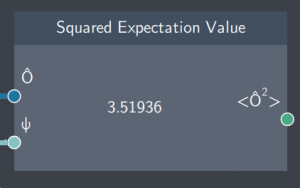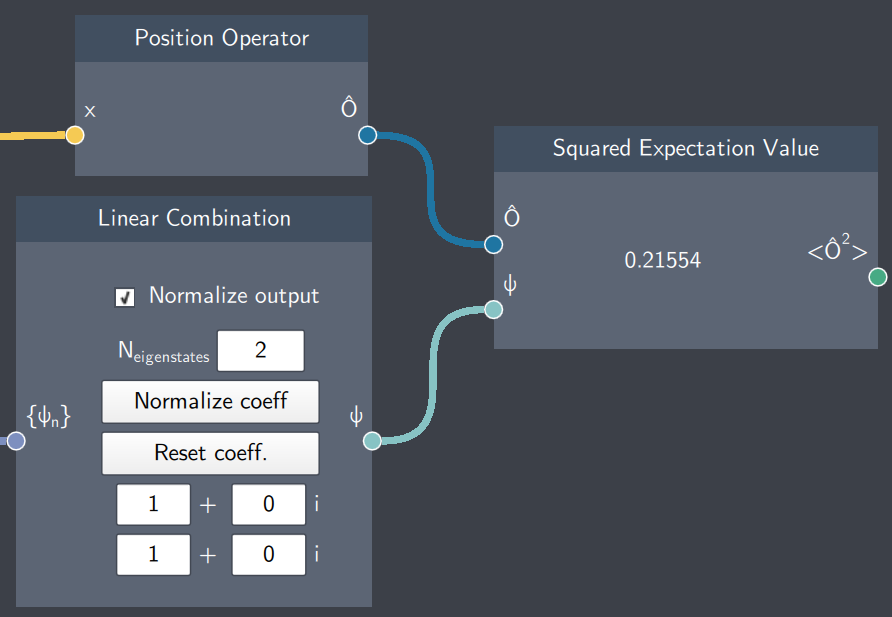# Squared Expectation Value

## Description

This node calculates the squared expectation value of any operator with regard to an arbitrary state. For an operator $\hat{O}$ and state $\psi$, this node calculates $\langle \psi | \hat{O}^2 | \psi \rangle$.## Inputs

The node has the following inputs:

• Operator ($\hat{O}$): Any operator defined in the Operator node such as $\hat{x}$, $\hat{p}$ or $\hat{H}$.
• State ($\psi$): Any state with regard to which the squared expecation value needs to be calculated.

## Content

The node displays the squared expectation value of the operator.

## Outputs

• Squared Expectation Value ($\langle \psi | \hat{O}^2 | \psi \rangle$): The node outputs the squared expectation value of the operator ($\hat{O}$) which is provided in the input.

## Example

In the example below, the squared expectation value of the position operator is calculated with regard to a non-stationary state and displayed in the node.